# Parallelograms - Same Base, Same Parallels

Go back to  'Triangles-Quadrilaterals'

Consider two parallelograms ABCD and ABEF, on the same base AB, and between the same parallels, as shown below: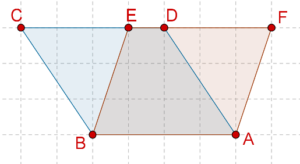What will be the relation between the areas of these two parallelograms?

Theorem: Parallelograms on the same base and between the same parallels are equal in area.

Proof: Consider the figure presented above. Can you see that $$\Delta BCE$$ and $$\Delta ADF$$ will be congruent? This is easy to show. We have:

1.  BC = AD (opposite sides of a parallelogram are equal)

2.  $$\angle BCE$$ = $$\angle ADF$$ (corresponding angles)

3.  $$\angle BEC$$ = $$\angle AFD$$ (corresponding angles)

By the ASA criterion, the two triangles are congruent, which means that their areas are equal. Now,

area(ABCD) = area(ABED) + area($$\Delta BCE$$)

Similarly,

area(ABEF) = area(ABED) + area($$\Delta ADF$$)

Clearly,

area(ABCD) = area(ABEF)

This completes the proof.

Next, consider a parallelogram ABCD and a rectangle ABEF on the same base and between the same parallels: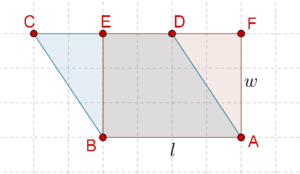Clearly, their areas will be equal. Now, the length and height (width) of the rectangle have been marked as l and w respectively. Therefore,

area(ABCD) = area(ABEF) = l × w

This means that the area of any parallelogram is equal to the product of its base and its height (the height of a parallelogram can be defined as the distance between its base and the opposite parallel).

Now, consider the following figure, which shows a parallelogram ABCD and a triangle ABE on the same base AB and between the same parallels: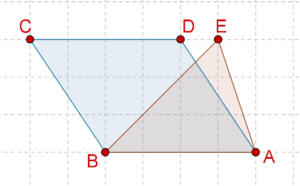What will be the relation between the areas of these two figures? Let us complete the parallelogram ABEF, as shown below: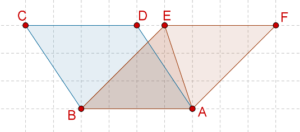Now, we have:

area($$\Delta ABE$$) = ½ area(ABFE)

= ½ area(ABCD)

Thus, the area of the triangle is exactly half of the area of the parallelogram. Let us define the height of a triangle as the distance between the base and the parallel through the opposite vertex. We can therefore say that the area of the triangle will be:

Area = ½ × base × height

This is shown below: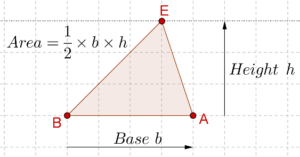Note that any of the three sides of the triangle can be taken as the base, but then the height will change accordingly.